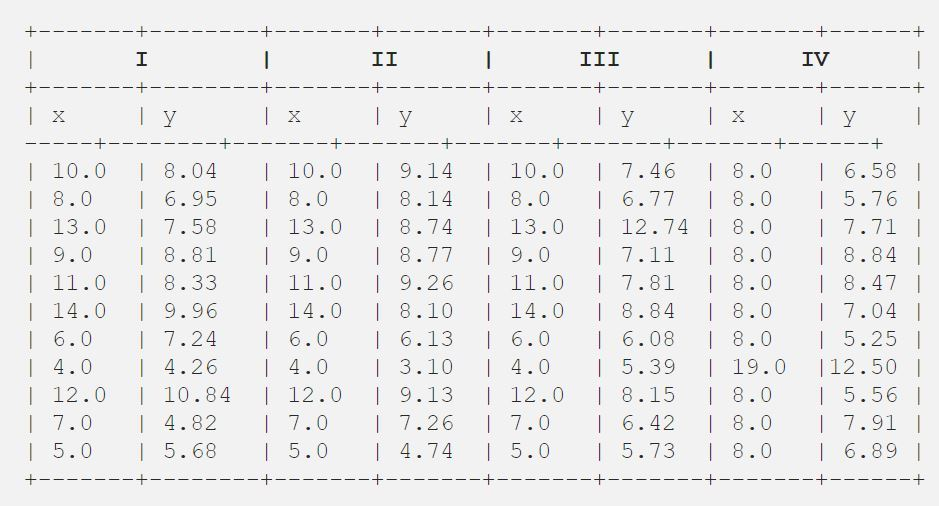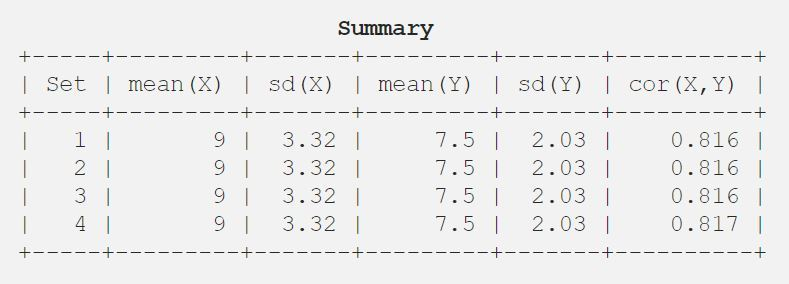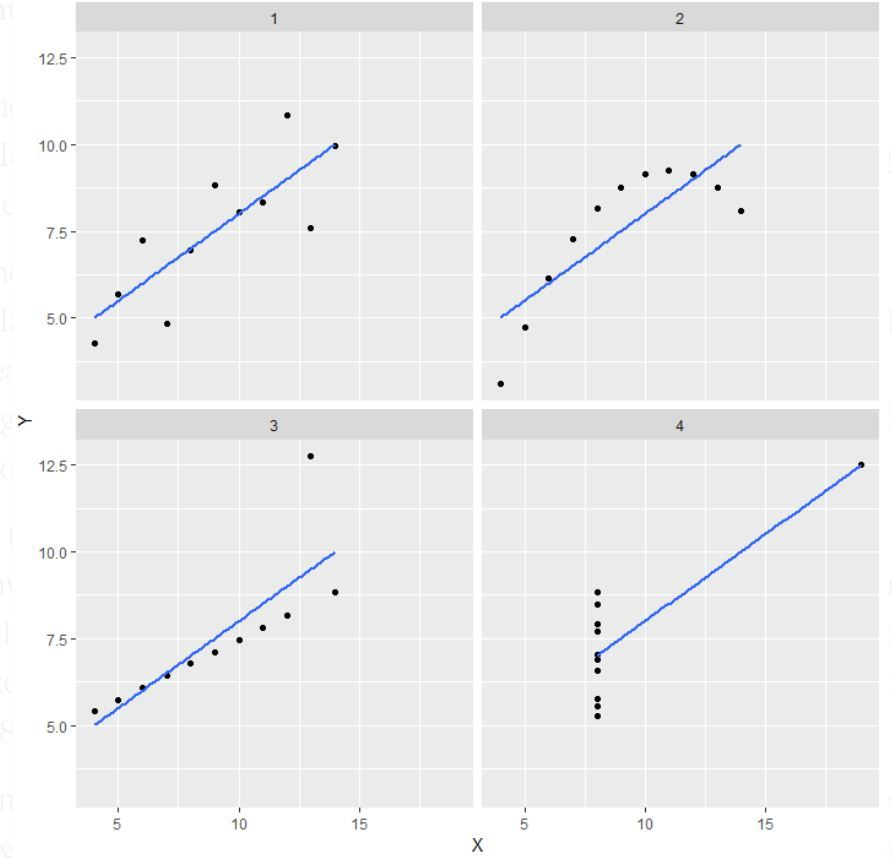# Anscombe’s quartet

• Last Updated : 16 Jul, 2020

According to the definition given in Wikipedia, Anscombe’s quartet comprises four datasets that have nearly identical simple statistical properties, yet appear very different when graphed. Each dataset consists of eleven (x,y) points. They were constructed in 1973 by the statistician Francis Anscombe to demonstrate both the importance of graphing data before analyzing it and the effect of outliers on statistical properties.

Simple understanding:
Once Francis John “Frank” Anscombe who was a statistician of great repute found 4 sets of 11 data-points in his dream and requested the council as his last wish to plot those points. Those 4 sets of 11 data-points are given below.After that, the council analyzed them using only descriptive statistics and found the mean, standard deviation, and correlation between x and y.

Code: Python program to find mean, standard deviation, and the correlation between x and y

 `# Import the required libraries``import` `pandas as pd``import` `statistics``from` `scipy.stats ``import` `pearsonr`` ` `# Import the csv file``df ``=` `pd.read_csv(``"anscombe.csv"``)`` ` `# Convert pandas dataframe into pandas series``list1 ``=` `df[``'x1'``]``list2 ``=` `df[``'y1'``]`` ` `# Calculating mean for x1``print``(``'%.1f'` `%` `statistics.mean(list1))`` ` `# Calculating standard deviation for x1``print``(``'%.2f'` `%` `statistics.stdev(list1))`` ` `# Calculating mean for y1``print``(``'%.1f'` `%` `statistics.mean(list2))`` ` `# Calculating standard deviation for y1``print``(``'%.2f'` `%` `statistics.stdev(list2))`` ` `# Calculating pearson correlation``corr, _ ``=` `pearsonr(list1, list2)``print``(``'%.3f'` `%` `corr)`` ` `# Similarly calculate for the other 3 samples`` ` `# This code is contributed by Amiya Rout`

Output:

```9.0
3.32
7.5
2.03
0.816
```

So let me show you the result in a tabular fashion for better understanding.Code: Python program to plot scatter plot

 `# Import the required libraries``from` `matplotlib ``import` `pyplot as plt``import` `pandas as pd`` ` `# Import the csv file``df ``=` `pd.read_csv(``"anscombe.csv"``)`` ` `# Convert pandas dataframe into pandas series``list1 ``=` `df[``'x1'``]``list2 ``=` `df[``'y1'``]`` ` `# Function to plot scatter``plt.scatter(list1, list2)`` ` `# Function to show the plot``plt.show()`` ` `# Similarly plot scatter plot for other 3 data sets`` ` `# This code is contributed by Amiya Rout`

For regression line refer this.
Output:Note: It is mentioned in the definition that Anscombe’s quartet comprises four datasets that have nearly identical simple statistical properties, yet appear very different when graphed.

Explanation of this output:

• In the first one(top left) if you look at the scatter plot you will see that there seems to be a linear relationship between x and y.
• In the second one(top right) if you look at this figure you can conclude that there is a non-linear relationship between x and y.
• In the third one(bottom left) you can say when there is a perfect linear relationship for all the data points except one which seems to be an outlier which is indicated be far away from that line.
• Finally, the fourth one(bottom right) shows an example when one high-leverage point is enough to produce a high correlation coefficient.

Application:
The quartet is still often used to illustrate the importance of looking at a set of data graphically before starting to analyze according to a particular type of relationship, and the inadequacy of basic statistic properties for describing realistic datasets.

My Personal Notes arrow_drop_up